### Home > GC > Chapter 7 > Lesson 7.3.1 > Problem7-105

7-105.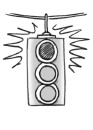Carolina compared her proof to that of Penn in problem 7-96. Like him, she wanted to prove that if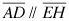and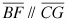, then a = d . Unfortunately, her statements were in a different order. Examine her proof below and help her decide if her statements are in a logical order in order to prove that a = d. Homework Help ✎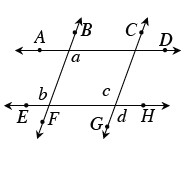Statements

Reasons

1.andGiven

2. a = b

If lines are parallel, alternate interior angles are equal.

3. a = c

Substitution

4. b = c

If lines are parallel, corresponding angles are equal.

5. c = d

Vertical angles are equal.

6. a = d

Substitution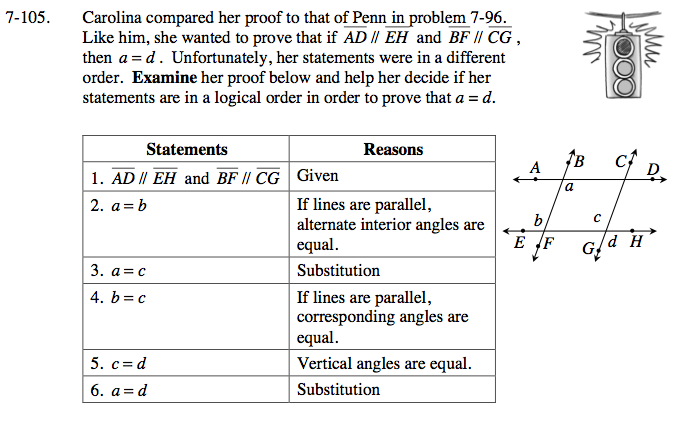Are all her statements in the proper order? Do the conclusions flow in a proper order?